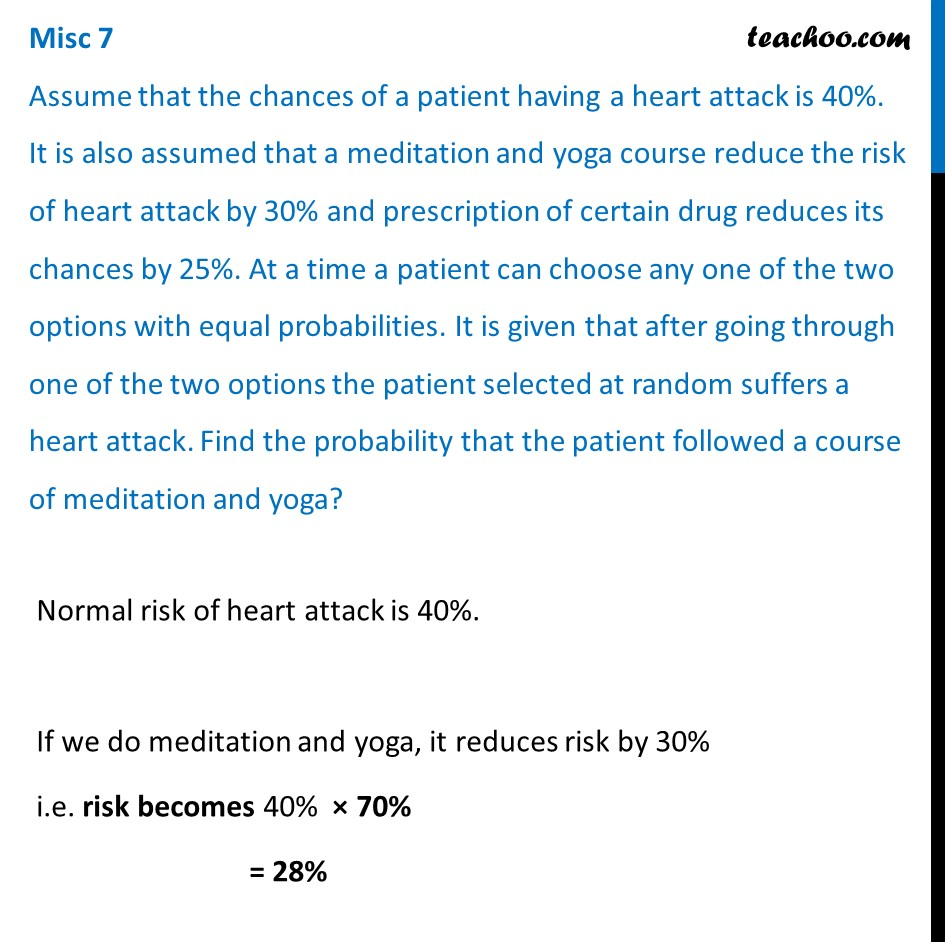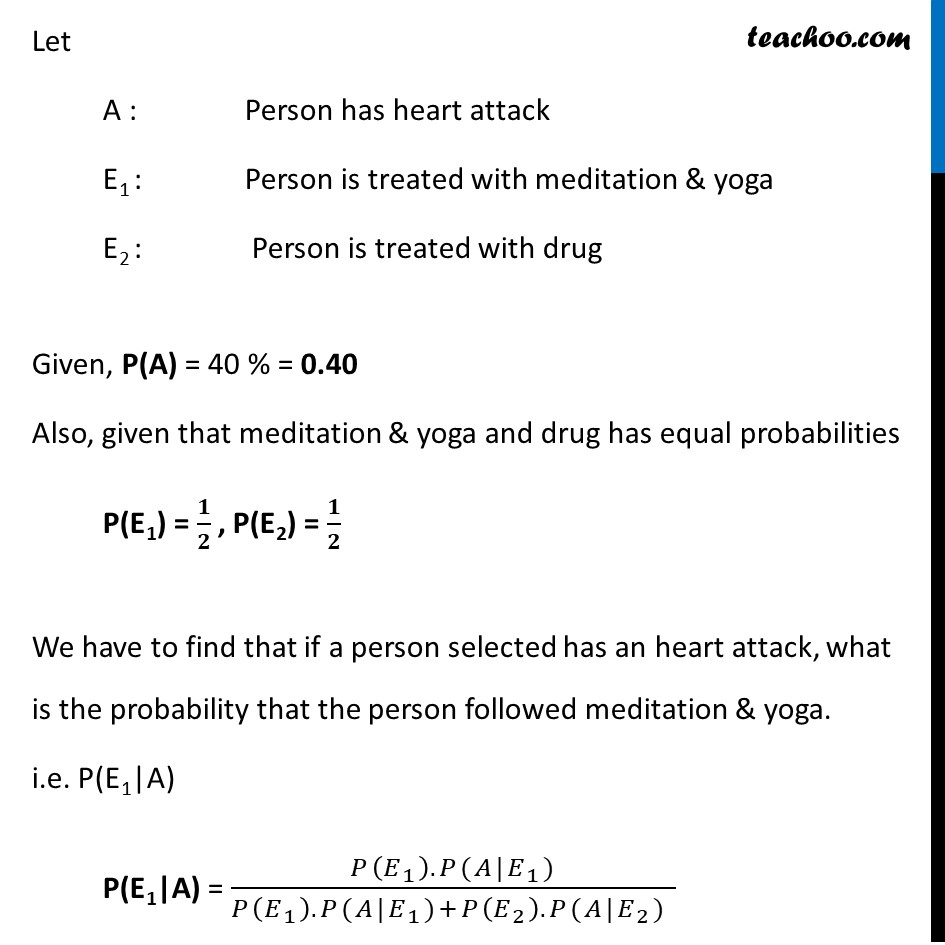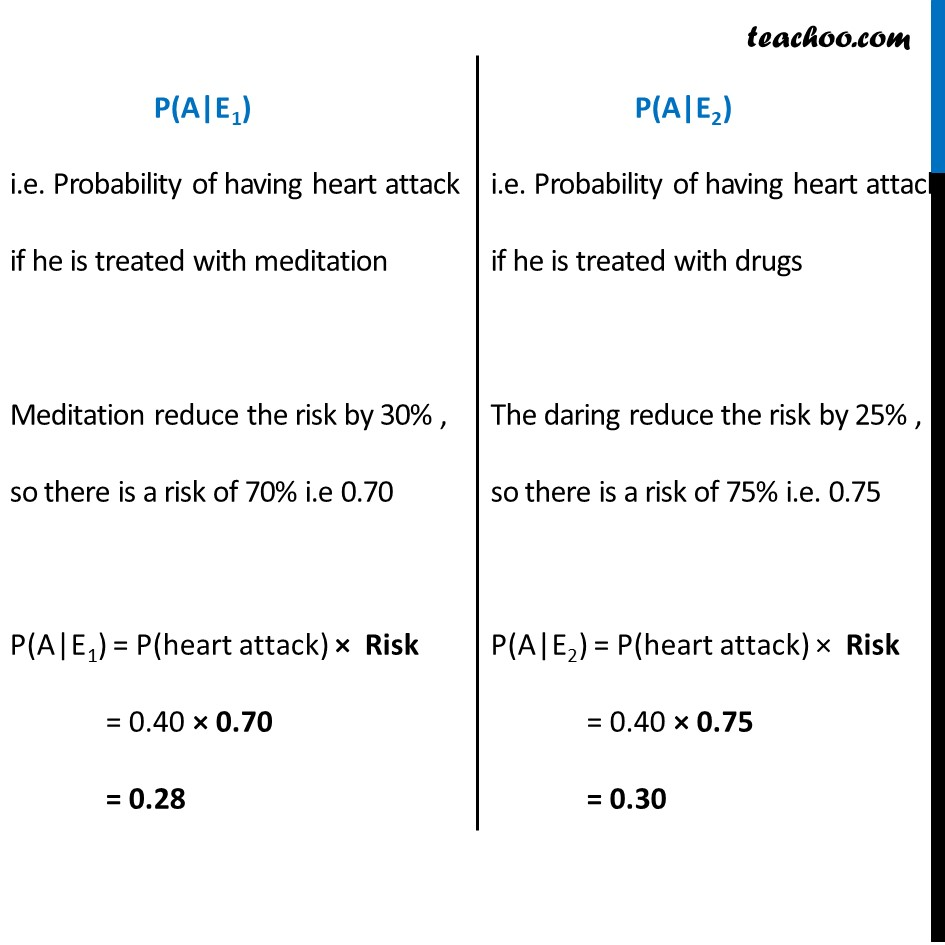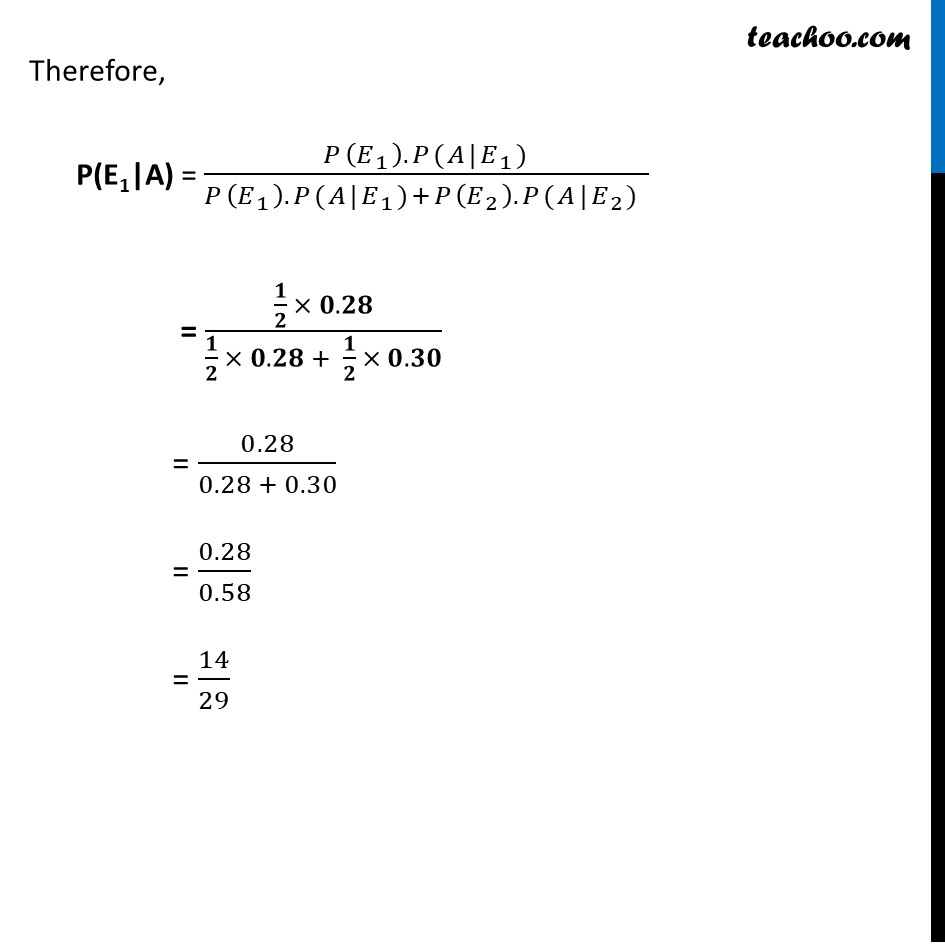Miscellaneous

Chapter 13 Class 12 Probability
Serial order wiseLearn in your speed, with individual attention - Teachoo Maths 1-on-1 Class

### Transcript

Misc 7 Assume that the chances of a patient having a heart attack is 40%. It is also assumed that a meditation and yoga course reduce the risk of heart attack by 30% and prescription of certain drug reduces its chances by 25%. At a time a patient can choose any one of the two options with equal probabilities. It is given that after going through one of the two options the patient selected at random suffers a heart attack. Find the probability that the patient followed a course of meditation and yoga?Normal risk of heart attack is 40%. If we do meditation and yoga, it reduces risk by 30% i.e. risk becomes 40% × 70% = 28% Let A : Person has heart attack E1 : Person is treated with meditation & yoga E2 : Person is treated with drug Given, P(A) = 40 % = 0.40 Also, given that meditation & yoga and drug has equal probabilities P(E1) = 𝟏/𝟐 , P(E2) = 𝟏/𝟐 We have to find that if a person selected has an heart attack, what is the probability that the person followed meditation & yoga. i.e. P(E1|A) P(E1|A) = (𝑃(𝐸_1 ).𝑃(𝐴|𝐸_1))/(𝑃(𝐸_1 ).𝑃(𝐴|𝐸_1)+𝑃(𝐸_2 ).𝑃(𝐴|𝐸_2) ) P(A|E1) i.e. Probability of having heart attack if he is treated with meditation Meditation reduce the risk by 30% , so there is a risk of 70% i.e 0.70 P(A|E1) = P(heart attack) × Risk = 0.40 × 0.70 = 0.28 P(A|E2) i.e. Probability of having heart attack if he is treated with drugs The daring reduce the risk by 25% , so there is a risk of 75% i.e. 0.75 P(A|E2) = P(heart attack) × Risk = 0.40 × 0.75 = 0.30 Therefore, P(E1|A) = (𝑃(𝐸_1 ).𝑃(𝐴|𝐸_1))/(𝑃(𝐸_1 ).𝑃(𝐴|𝐸_1)+𝑃(𝐸_2 ).𝑃(𝐴|𝐸_2) ) = (𝟏/𝟐 × 𝟎.𝟐𝟖)/(𝟏/𝟐 × 𝟎.𝟐𝟖 + 𝟏/𝟐 × 𝟎.𝟑𝟎) = 0.28/(0.28 + 0.30) = 0.28/0.58 = 14/29